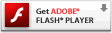# Interactive Generative Art Series – 04 – anchorThanks for keeping up with this random generative art series. I know I’m having a ball just playing with code one step at a time. To watch each iteration, I was getting tired of drawing with my mouse to see the generative art, and as others had pointed out, in order for it to truly be generative, it shouldn’t depend on me moving my mouse. So instead of using the mouse position as my target anchor, I created a new node named anchor. This anchor I’m animating with simple random brownian motion which I’ve blogged about before. It just meanders along the stage as it pleases and the other ball will “chase” it just like it chased your mouse in the previous examples. The part that gets exciting is that my anchor wraps from one side of the stage to the other, while the ball does not. One second it’s target is far right and the next it switches to far left, this makes for some really interesting paths and lines. Experiment with the velocity of the anchor in lines 47 + 48 to see interesting effects. One thing I’m beginning to notice however is the performance of the little app gets a bit slower the longer you let it run, perhaps I didn’t realize it before since I didn’t have the attention to keep moving my mouse and watching it follow, now that it truly is generative (and less interactive btw), I watch it longer (seems kind of backwards to what I’d expect).

Note: in this example I have the anchor ball visible, as well as the other ball, but for an actual production, I’d have them both hidden and the lines just appearing. I prefer it that way but though you’d better see what was actually happening with them visible, even though it kills some of the magic. It’s like watching a magic trick when you already know how it’s done.### 04 Anchor, play here

Please visit the blog article to view this interactive flash content. Flash plug-in required:### actionscript source code

[cc lang=”actionscript”]
var ball:Sprite = new Sprite();
ball.graphics.beginFill(0x000000, 0);
ball.graphics.drawCircle(0, 0, 5);
ball.graphics.endFill();

var anchor:Sprite = new Sprite();

anchor.graphics.beginFill(0x333333, 0);
anchor.graphics.drawCircle(0, 0, 12);
anchor.graphics.endFill();

var div:Number = .1;
var line_max_width:Number = 42;
var line_min_width:Number = 2;
var line_width:Number = randomRange(line_min_width, line_max_width);
var line_width_velocity:Number = 0;
var dampen:Number = 0.95;

var ballax:Number = 0;
var ballay:Number = 0;
var oldx:Number = ball.x;
var oldy:Number = ball.y;

var anchorvx:Number = 0;
var anchorvy:Number = 0;

anchor.x = stage.stageWidth/2;
anchor.y = stage.stageHeight/2;

var colors:Object = new Object();
colors.r = 0;
colors.g = 122;
colors.b = 255;
colors.rv = 0;
colors.gv = 0;
colors.bv = 0;
colors.min = 0;
colors.max = 200;
var color_first:Number = 0xFFFFFF;
var color_second:Number = rgb2hex(colors.r, colors.g, colors.b);

function loop ()
{
oldx = ball.x;
oldy = ball.y;

anchorvx += randomRangeAxis(2);
anchorvy += randomRangeAxis(2);

anchor.x += anchorvx;
anchor.y += anchorvy;

anchorvx *= dampen;
anchorvy *= dampen;

if(anchor.x > stage.stageWidth) {
anchor.x = 0 – anchor.width;
}
else if(anchor.x < 0 – anchor.width) { anchor.x = stage.stageWidth; } if(anchor.y > stage.stageHeight) {
anchor.y = 0 – anchor.height;
}
else if(anchor.y < 0 – anchor.height) { anchor.y = stage.stageHeight; } ball.x -= ballax = (ballax + (ball.x – anchor.x) * div) * .9; ball.y -= ballay = (ballay + (ball.y – anchor.y) * div) * .9; line_width_velocity += randomRangeAxis(1); line_width += line_width_velocity; line_width_velocity *= dampen; if(line_width > line_max_width) {
line_width = line_max_width;
line_width_velocity = 0;
}
else if (line_width < line_min_width) {
line_width = line_min_width;
line_width_velocity = 0;
}

color_step();
color_first = color_second;
color_second = rgb2hex(colors.r, colors.g, colors.b);

var dx:Number = ball.x – oldx;
var dy:Number = ball.y – oldy;
this.graphics.lineStyle(line_width);
this.graphics.lineTo(ball.x, ball.y);
}

setInterval(loop, 1000/30);
function rgb2hex(r:Number, g:Number, b:Number):Number {
return(r<<16 | g<<8 | b); } function color_step(){ colors.rv += Math.random() * 20 – 10; colors.r += colors.rv; colors.rv *= dampen; if (colors.r > colors.max) {
colors.r = colors.max;
} else if (colors.r < colors.min){ colors.r = colors.min; } colors.gv += Math.random() * 20 – 10; colors.g += colors.gv; colors.gv *= dampen; if (colors.g > colors.max) {
colors.g = colors.max;
} else if (colors.g < colors.min){ colors.g = colors.min; } colors.bv += Math.random() * 20 – 10; colors.b += colors.bv; colors.bv *= dampen; if (colors.b > colors.max) {
colors.b = colors.max;
} else if (colors.b < colors.min){
colors.b = colors.min;
}
}
//random number between min and max
function randomRange(max:Number, min:Number = 0):Number {
return Math.random() * (max – min) + min;
}
//random number range centered at 0 with the specified max, randomRange(-max, max)
function randomRangeAxis(max:Number):Number {
return Math.random() * (max * 2) – max;
}

[/cc]